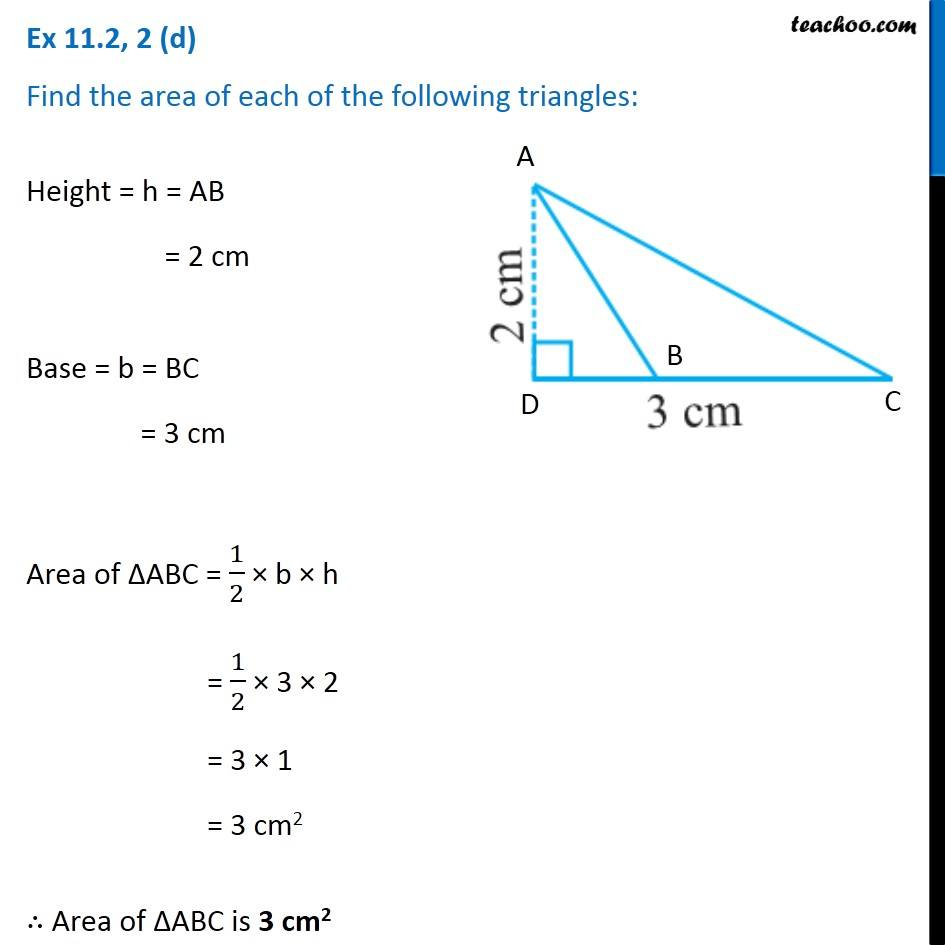Ex 11.2

Chapter 11 Class 7 Perimeter and Area
Serial order wiseGet live Maths 1-on-1 Classs - Class 6 to 12

### Transcript

Ex 11.2, 2 (d) Find the area of each of the following triangles: Height = h = AB = 2 cm Base = b = BC = 3 cm Area of ∆ABC = 1/2 × b × h = 1/2 × 3 × 2 = 3 × 1 = 3 cm2 ∴ Area of ∆ABC is 3 cm2